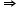Chemistry
Easy

Question

# Oxidation number of Fe in Fe2O3:

## 0- 2+ 2+ 3Hint:

## The correct answer is: + 3

### For calculating the oxidation number of any element in a compound first we've to see whether the compound is neutral or charged. The total summation of oxidation numbers in the compound is equal to that. We consider the asked oxidation number to be unknown and the other elements' oxidation number we put accordingly.Here in Fe2O3, oxidation number of oxygen is -2 and oxidation number of iron is unknown.2(x) + 3(-2) = 0 as the compound is neutral and x is the oxidation number of iron.x = +3Hence, oxidation number of Fe is +3.#### With Turito Foundation.#### Get an Expert Advice From Turito.